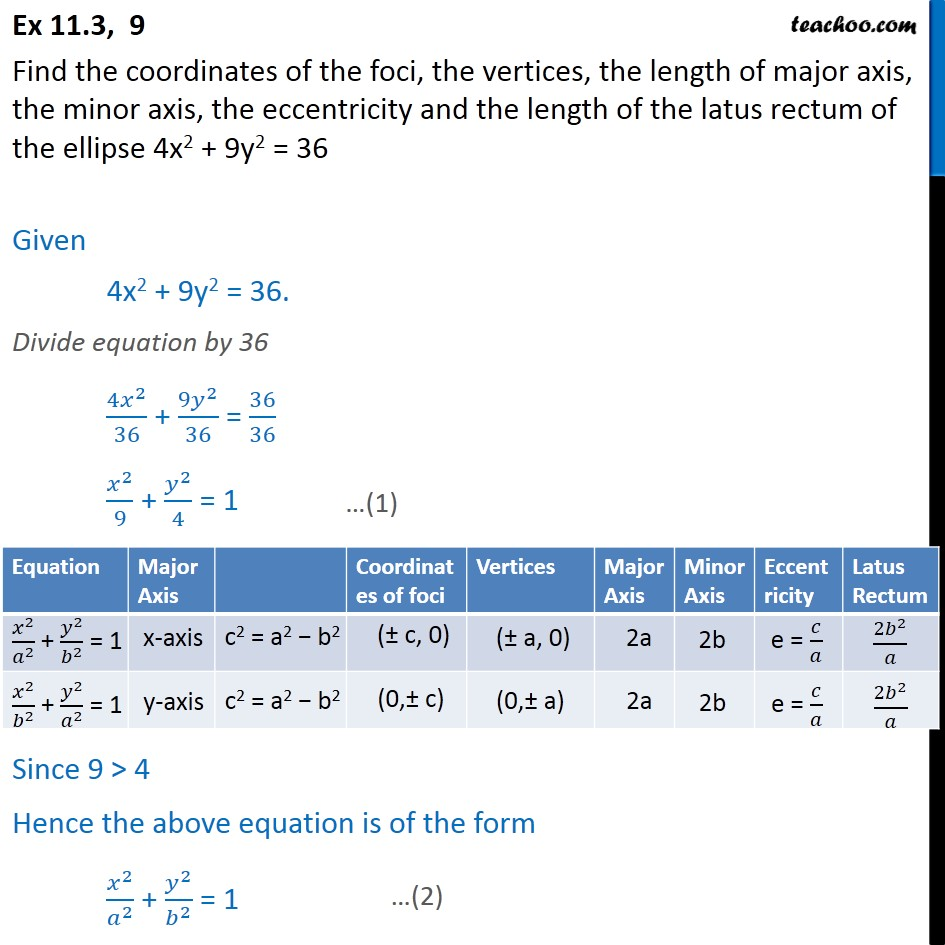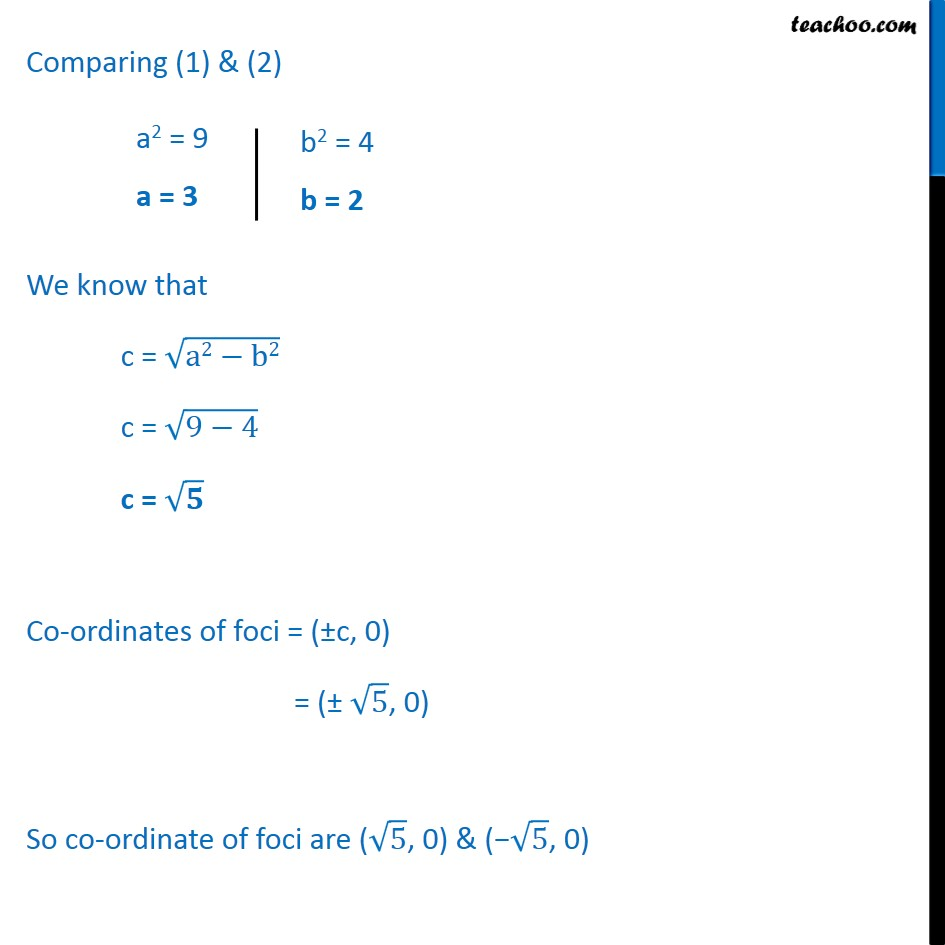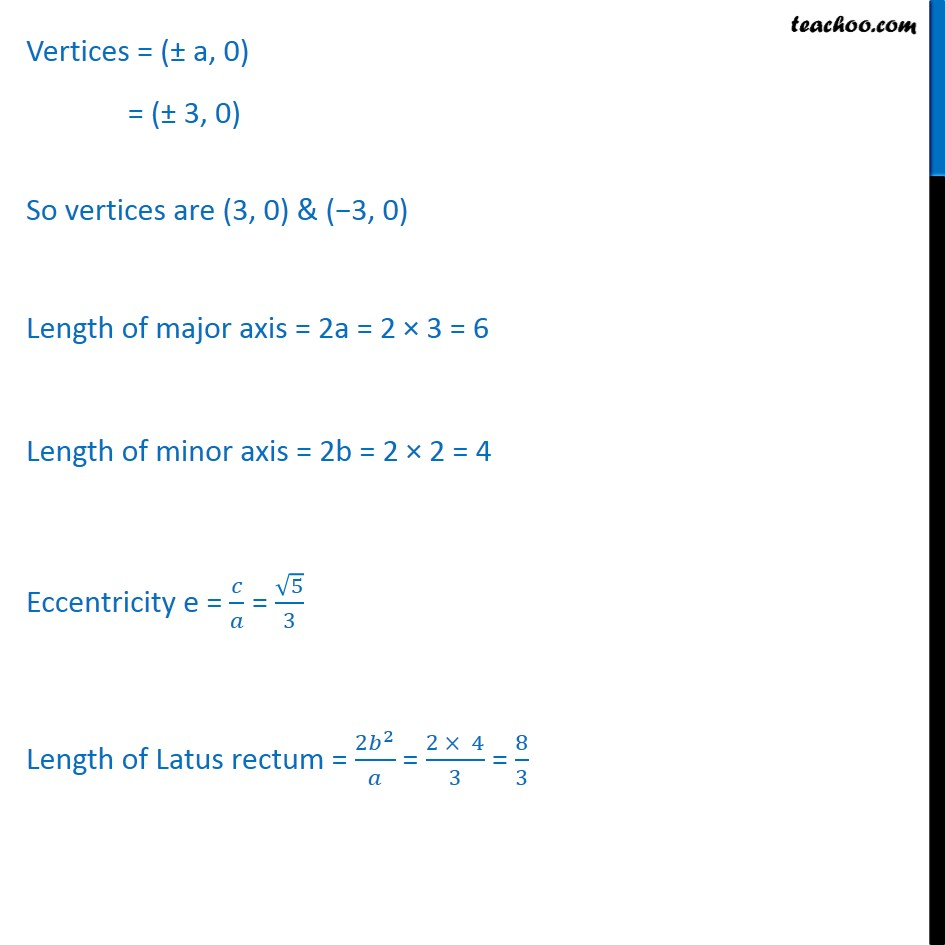Ex 11.3

Chapter 11 Class 11 Conic Sections (Term 2)
Serial order wise### Transcript

Ex 11.3, 9 Find the coordinates of the foci, the vertices, the length of major axis, the minor axis, the eccentricity and the length of the latus rectum of the ellipse 4x2 + 9y2 = 36 Given 4x2 + 9y2 = 36. Divide equation by 36 4 2 36 + 9 2 36 = 36 36 2 9 + 2 4 = 1 Since 9 > 4 Hence the above equation is of the form 2 2 + 2 2 = 1 Comparing (1) & (2) We know that c = a2 b2 c = 9 4 c = Co-ordinates of foci = ( c, 0) = ( 5 , 0) So co-ordinate of foci are ( 5 , 0) & ( 5 , 0) Vertices = ( a, 0) = ( 3, 0) So vertices are (3, 0) & ( 3, 0) Length of major axis = 2a = 2 3 = 6 Length of minor axis = 2b = 2 2 = 4 Eccentricity e = = 5 3 Length of Latus rectum = 2 2 = 2 4 3 = 8 3